# Representation of Real Numbers on Number Line

Representation of Real Numbers on Number Line
Go back to  'Coordinate Geometry'

Would you come along with us to walk through the number line and explore the world of infinite numbers?

Now, if you are asked to pick up the most commonly used numbers and store them in your bag, you would probably begin with picking the natural numbers followed by the integers.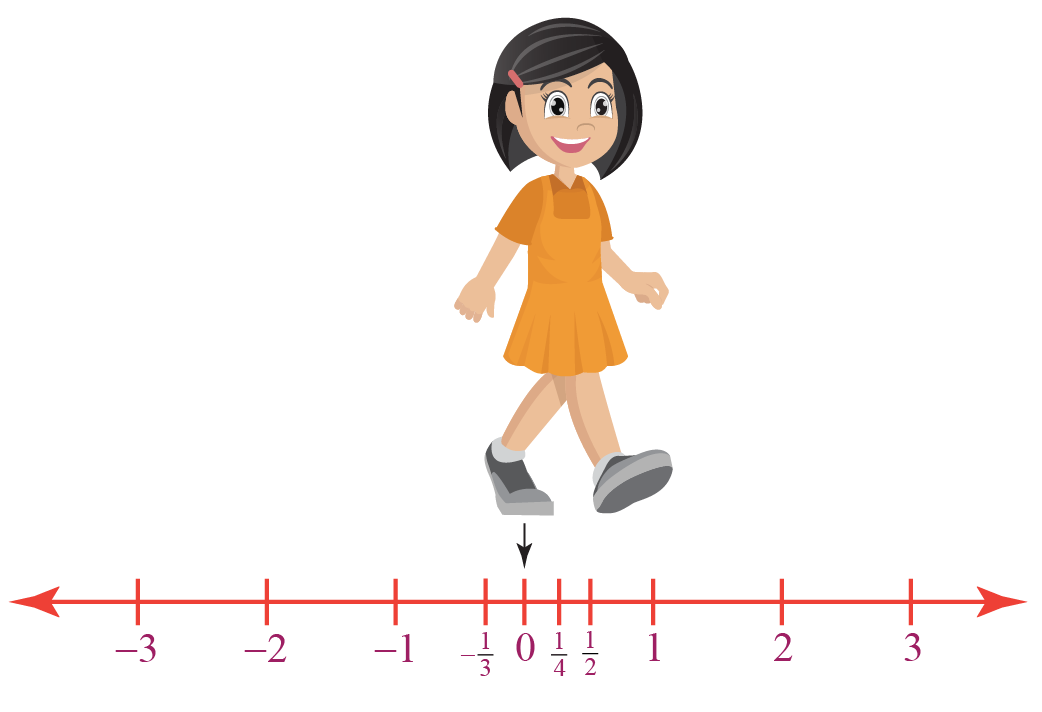Are there any numbers left now?

Of course! We have rational numbers and irrational numbers too.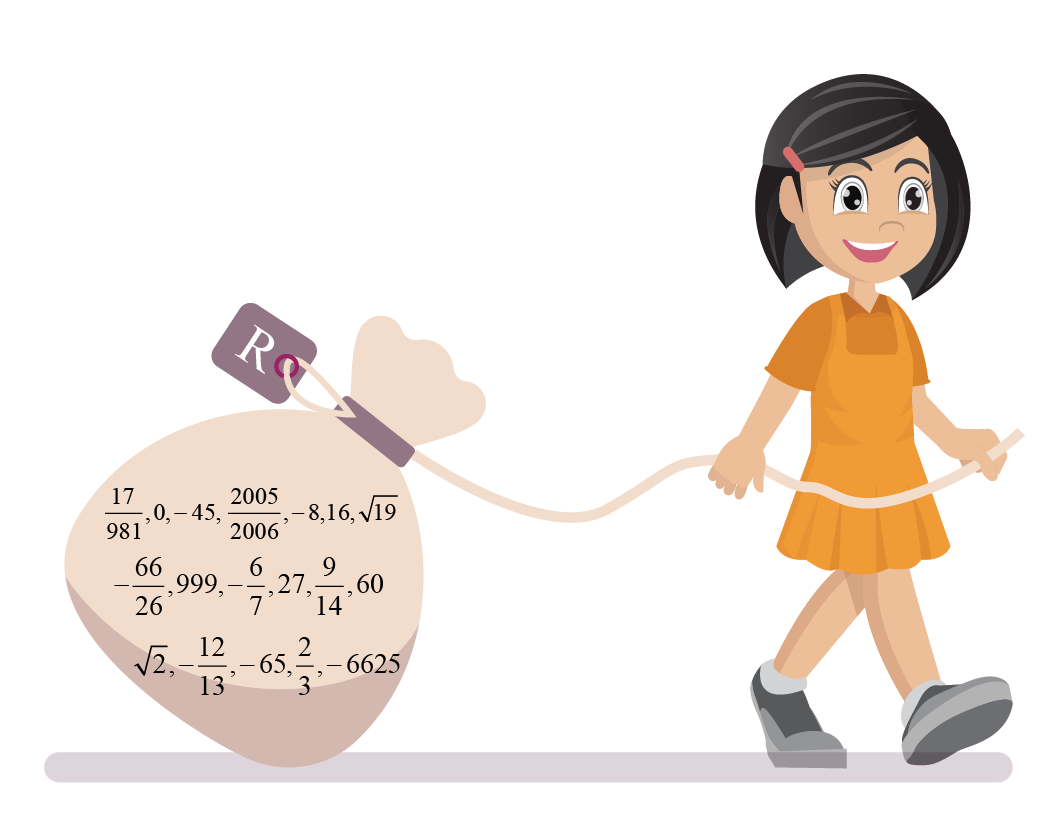Now, will there be any number left on the number line?

The answer is no! The collection of numbers in your bag now has the set of real numbers denoted by $$\mathbb{R}$$

In this mini-lesson, we will explore the world of real line. We will learn the real number line definition, graphing real numbers, real number line graph, graphing rational numbers on a number line, graphing irrational numbers on a number line, graphing whole numbers on a number line, and discover the other interesting aspects of it. You can try your hand at solving a few interesting interactive questions at the end of the page.

## Lesson Plan

 1 What Are Real Numbers? 2 Important Notes 3 Solved Examples 4 Thinking Out of the Box! 5 Interactive Questions

## What Are Real Numbers?

### Real Numbers Definition

• The set of real numbers is the union of the set of rational numbers $$\mathbb{Q}$$ and the set of irrational numbers $$\mathbb{I}$$.
• The set of real numbers is denoted by $$\mathbb{R}$$.
 $$\mathbb{R}=\mathbb{Q} \cup \mathbb{I}$$

This indicates that real numbers include natural numbers, whole numbers, integers, rational numbers, and irrational numbers.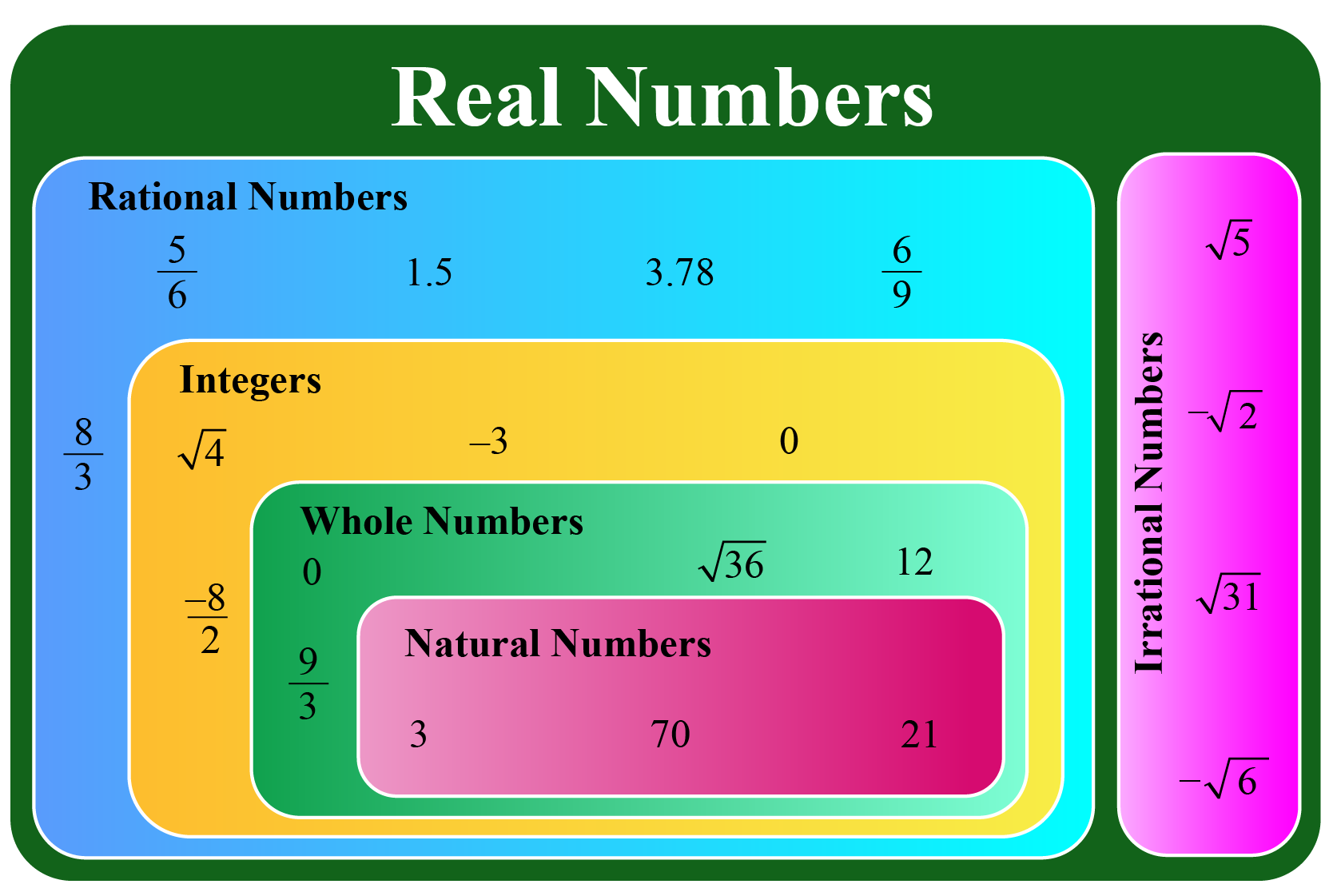This leads us to the next question; which numbers are NOT real numbers?

The numbers that are neither rational nor irrational, say $$\sqrt{-1}$$, are NOT real numbers.

These numbers include the set of complex numbers, $$\mathbb{C}$$.

We can understand this better from the following table:

Number Set Is it a part of the set of real numbers?

Natural Numbers

Whole Numbers

Integers

Rational Numbers

Irrational Numbers

Complex Numbers

## What Do You Mean By Real Number Line?

### Real Number Line Definition

Now you already know that a real number can be a rational number or an irrational number.

So, we can say that every real number can be represented by a unique point on a number line.

Every point on the number line shows a unique real number.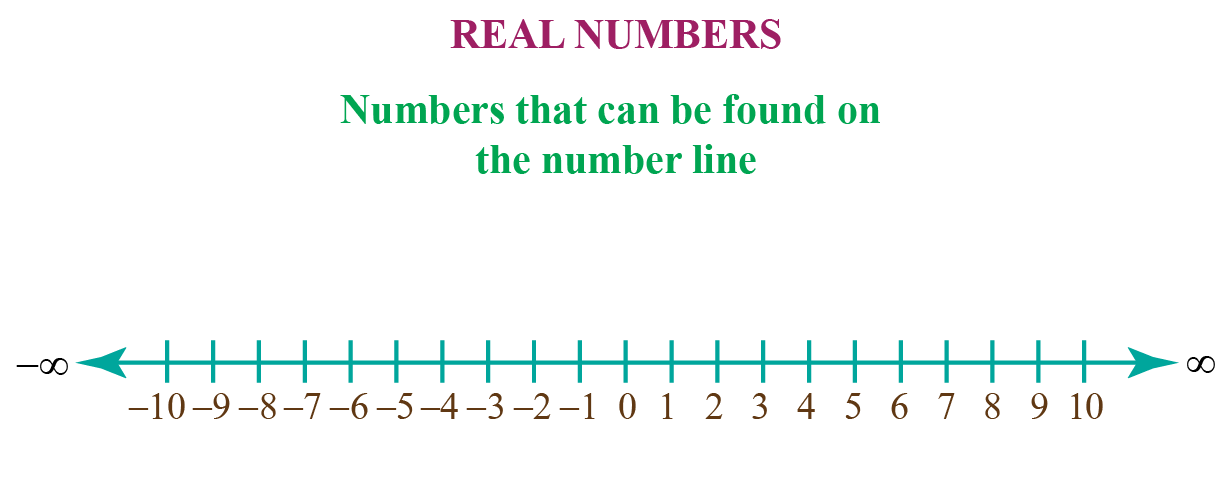## How To Represent Real Numbers on a Number Line?

You already know how to graph whole numbers on a number line.

A number line helps you to display real numbers by representing them by a unique point on the line.

When we represent a real number by a point, the point is called a coordinate.

When we represent the point on a real number line representing a coordinate, the real line is called its graph.

### Real Number Line Graph

Note the following steps to represent real numbers on a number line:

1. Draw a horizontal line with arrows on both ends and mark off the number 0 anywhere. The number 0 is called the origin.
2. Mark off the equal length on both sides of the origin and label it with a definite scale.
3. Remember that the positive numbers lie on the right side of the origin and the negative numbers lie on the left side of the origin.

Observe the numbers highlighted on the number line.

It shows the real numbers $$-\dfrac{5}{2}$$, 0, $$\dfrac{3}{2}$$ and 2.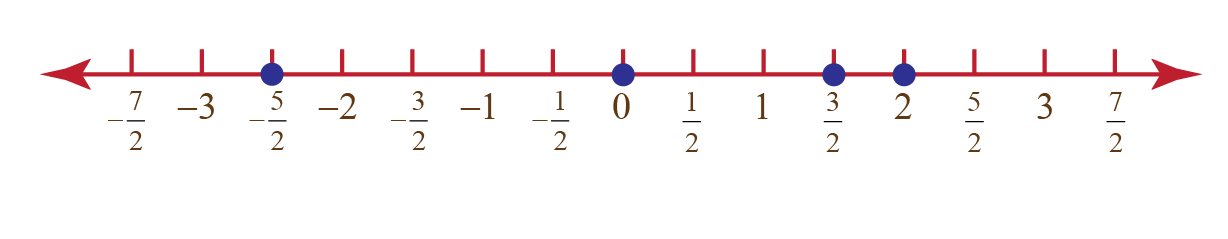Here is a simulation for you to plot any real number on a number line.

Just give any real number between -5 and 5 and click on the 'GO' button to check how it is represented on the number line.

## Solved Examples

 Example 1

Emma was asked to identify the real numbers in the following list of numbers:

$\sqrt{6}, -3, 3.15, \frac{-1}{3}, \sqrt{-5}$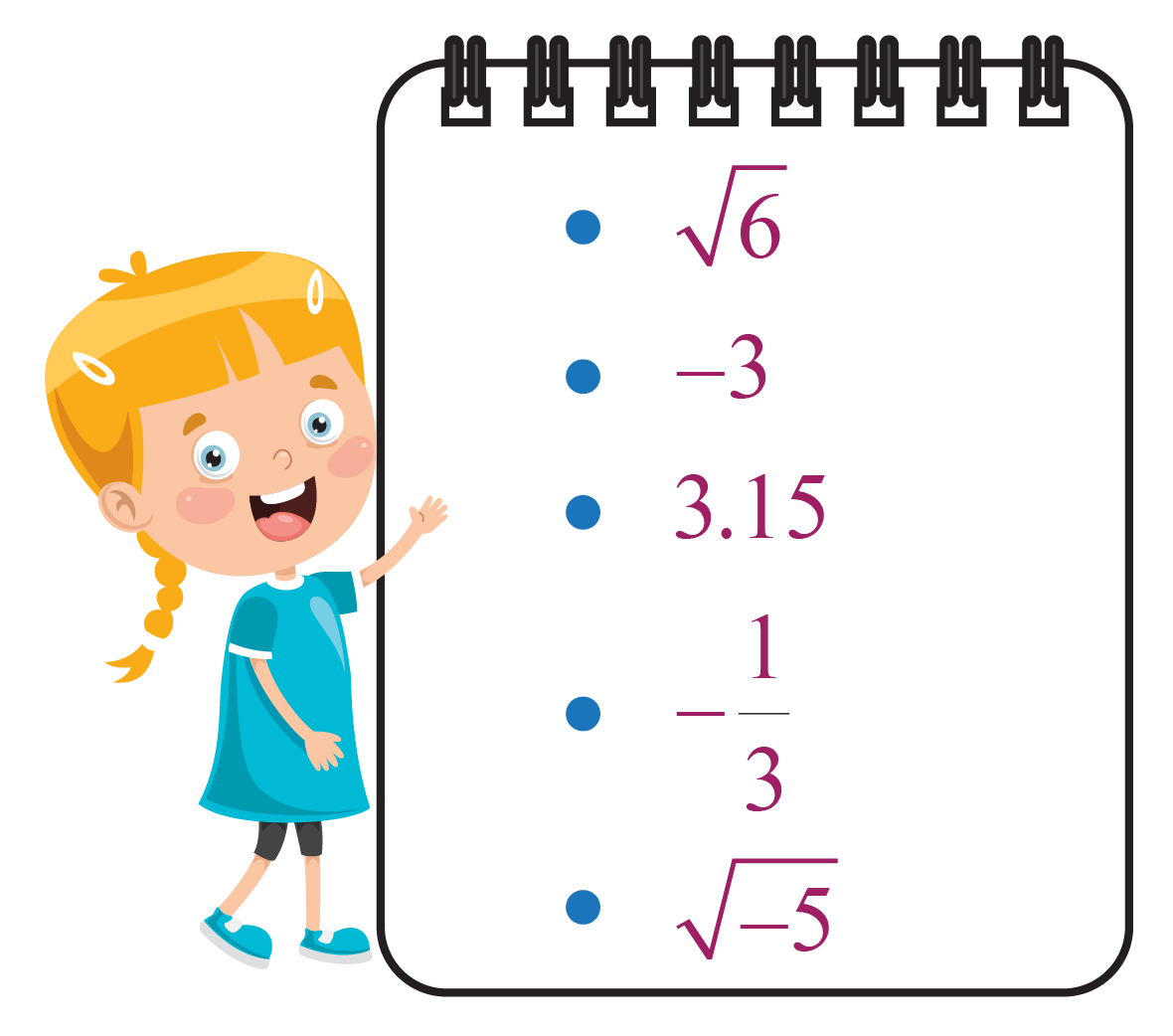Can you help her?

Solution

Among the given numbers, $$\sqrt{-5}$$ is a complex number. Hence, it cannot be a real number.

The other numbers are either rational or irrational. Thus, they are real numbers.

 $$\therefore$$ $$\ \sqrt{6}, -3, 3.15 \text{ and } \frac{-1}{3}$$
 Example 2

Is $$\sqrt{2}$$ a real number?

What type of real number is it?

Can you plot it on a number line?

Solution

Yes, $$\sqrt{2}$$ is a real number.

It is categorized as an irrational number.

Let's locate $$\sqrt{2}$$ on a number line and name the vertices of the square as shown.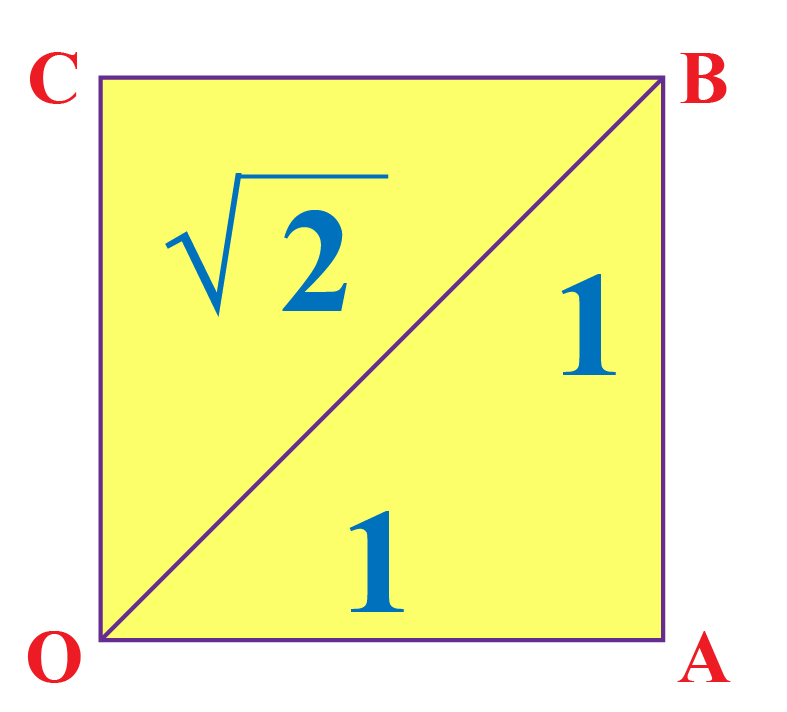Keep the vertex $$O$$ at zero.

By Pythagoras Theorem, $$OB=\sqrt{2}$$

Using a compass with center $$O$$ and radius $$OB$$, draw an arc intersecting the number line at point $$P$$.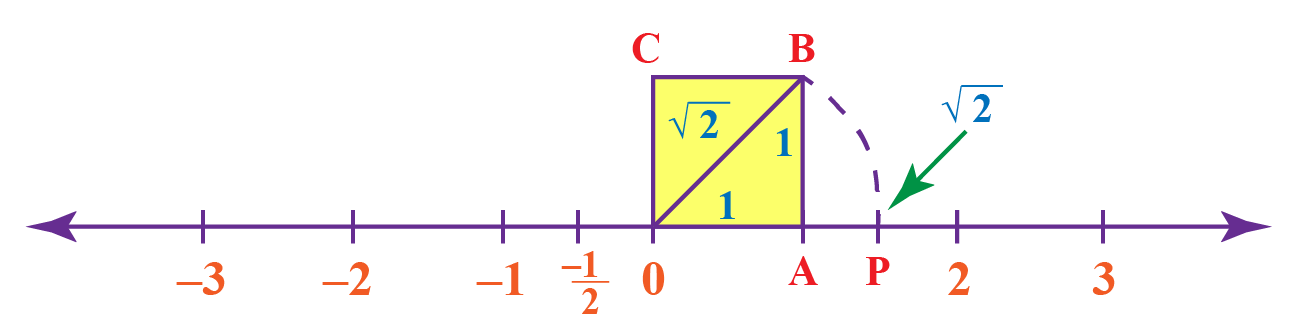The point $$P$$ corresponds to $$\sqrt{2}$$ on the number line.

 $$\therefore$$ The number $$\sqrt{2}$$ is shown on the number line.
 Example 3

Sam's teacher plotted the numbers $$\dfrac{1}{2}$$ and $$\dfrac{2}{3}$$ on the number line.

She asked Sam to find and plot a rational number between these two numbers on the number line.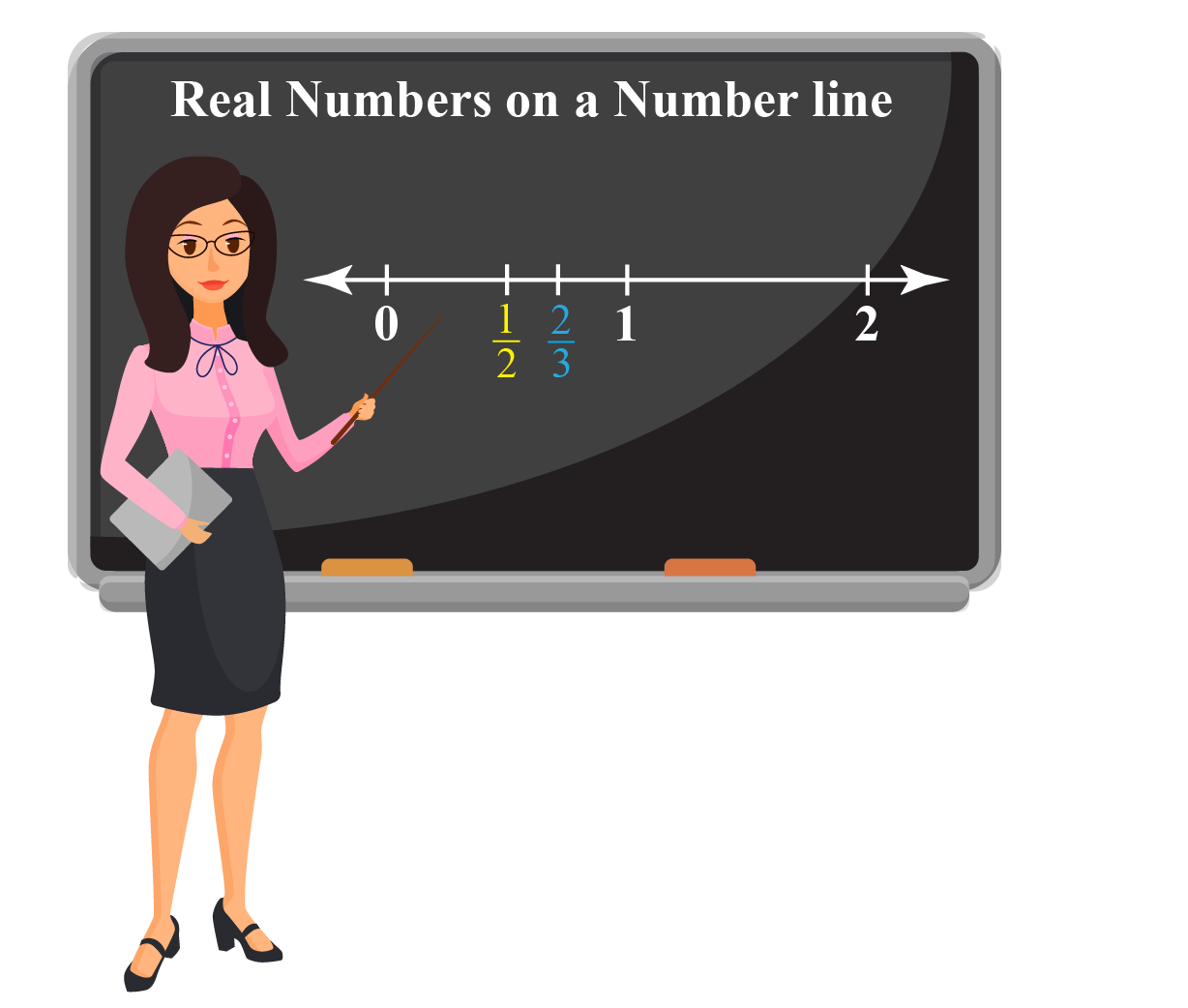Can you help Sam plot a rational number between them?

Solution

We know that the midpoint of any two numbers lies between the two numbers.

Let's find the midpoint of the given two rational numbers.

\begin{aligned} \dfrac{ \dfrac{1}{2}+ \dfrac{2}{3}}{2} &= \dfrac{\dfrac{3}{6}+ \dfrac{4}{6}}{2}\\[0.3cm] &= \dfrac{ \left(\dfrac{7}{6} \right)}{2}\\[0.3cm] &= \dfrac{ \left(\dfrac{7}{6} \right)}{ \left(\dfrac{2}{1} \right)}\\[0.3cm] &= \dfrac{7}{6} \times \dfrac{1}{2}\\[0.3cm] &= \dfrac{7}{12} \end{aligned}

This rational number $$\dfrac{7}{12}$$ is shown on the following number line.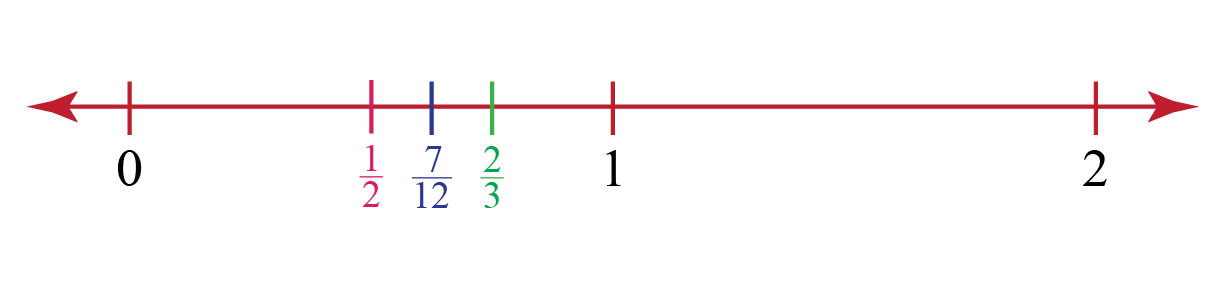$$\therefore$$ The rational number is $$\dfrac{7}{12}$$Think Tank
1. Can you prove that $$\sqrt{2}$$ is an irrational number using contradiction?
2. Can you plot $$\sqrt{5}$$ on a number line?

## Interactive Questions

Here are a few activities for you to practice.

Select/type your answer and click the "Check Answer" button to see the result.

## Let's Summarize

The mini-lesson targeted the fascinating concept of Representation of Real Numbers on a Number Line. The math journey around Representation of Real Numbers on a Number Line starts with what a student already knows, and goes on to creatively crafting a fresh concept in the young minds. Done in a way that not only it is relatable and easy to grasp, but also will stay with them forever. Here lies the magic with Cuemath.

We hope you enjoyed learning graphing real numbers on a real line through this lesson.

## About Cuemath

At Cuemath, our team of math experts is dedicated to making learning fun for our favorite readers, the students!

Through an interactive and engaging learning-teaching-learning approach, the teachers explore all angles of a topic.

Be it worksheets, online classes, doubt sessions, or any other form of relation, it’s the logical thinking and smart learning approach that we, at Cuemath, believe in.

## FAQs on Representation of Real Numbers on a Number Line

### 1. How do you identify real numbers on a number line?

All numbers on a number line are real number numbers. Therefore, it is called a real line.

### 2. How do you classify any number?

Refer to the tree diagram shown below to classify any number.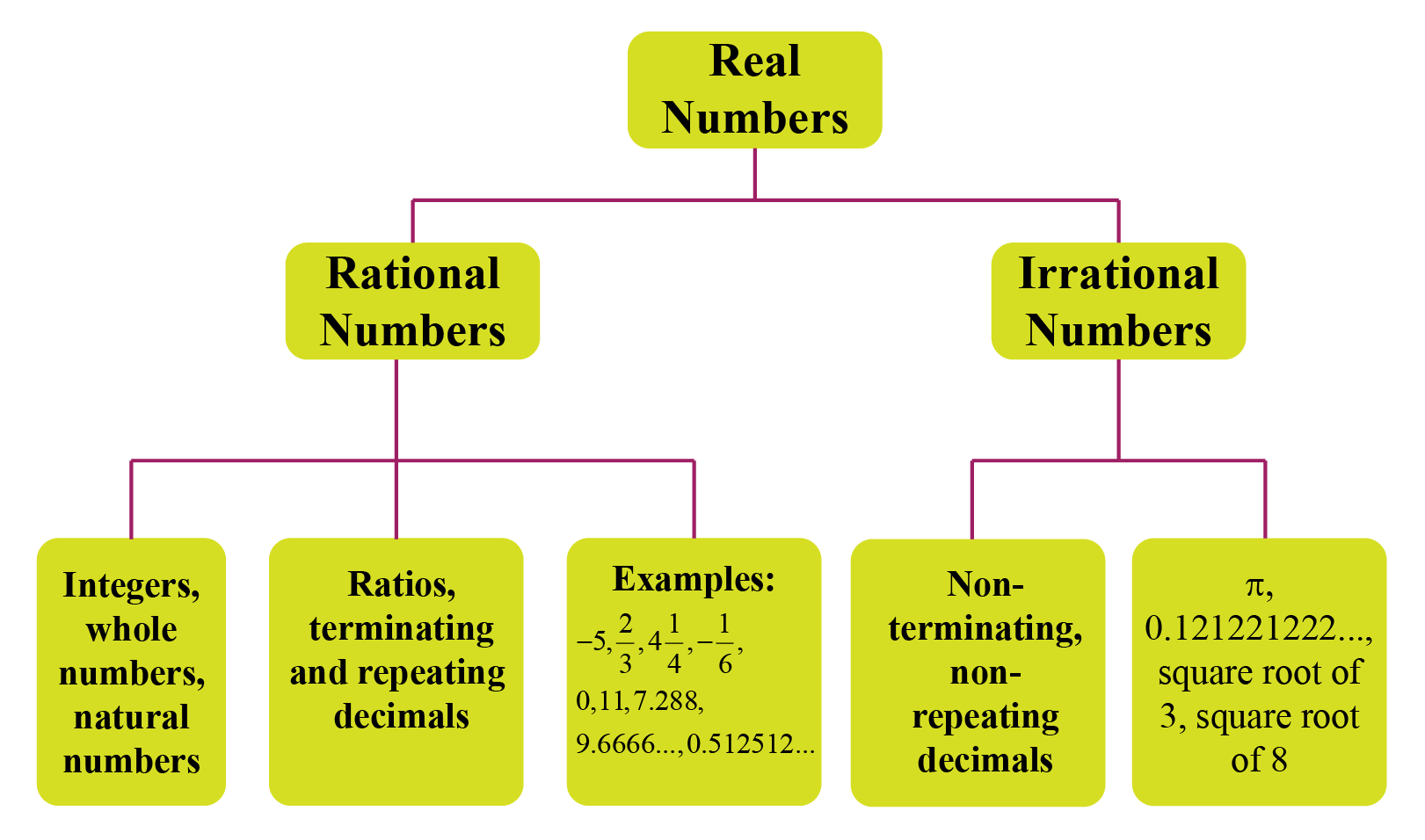### 3. How do you graph points on a number line?

Note the following steps to represent real numbers on a number line:

1. Draw a horizontal line with arrows on both ends and mark off the number 0 anywhere. The number 0 is called the origin.
2. Mark off the equal length on both sides of the origin and label it with a definite scale.
3. Remember that the positive numbers lie on the right side of the origin and the negative numbers lie on the left side of the origin.

### 4. How do you plot fractional numbers on a number line?

Observe the following steps to represent fractional numbers on a number line:

1. Draw a horizontal line with arrows on both ends and mark off the number 0 anywhere. The number 0 is called the origin.
2. Mark off the equal length on both sides of the origin and label it with a definite scale.
3. Remember that the positive fractions lie on the right side of the origin and the negative fractions lie on the left side of the origin.

The following number line shows a few fractions marked on it.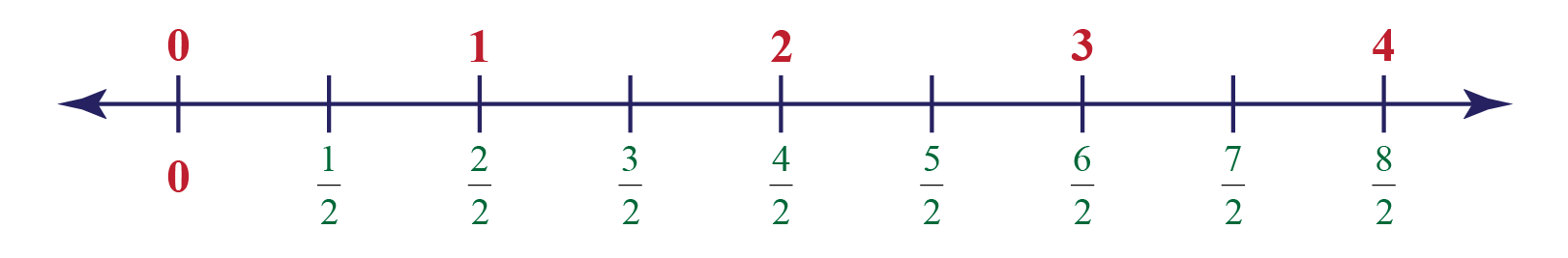### 5. How do you tell if a square root is a real number?

If the number inside the $$\sqrt{ }$$ symbol is positive, then it is a real number. For example, $$\sqrt{2}$$ is a real number.

If the number inside the $$\sqrt{ }$$ symbol is negative, then it is not a real number. For example, $$\sqrt{-2}$$ is not a real number.

### 6. Is Pi a real number?

Yes, $$\pi$$ is a real number.

### 7. Does a real line and number line mean the same?

Yes, a number line is also called a real line.

### 8. Is the real number line connected?

Yes, the real number line is connected because if we take any two points on the real line, the line segment joining the points will lie within the real line.

### 9. What is the set of all real numbers?

The set of real numbers is a set containing all the rational and irrational numbers.

### 10. Can we represent all real numbers on a number line?

Yes, we can represent all real numbers on a number line.

Download Coordinate Geometry Worksheets
Coordinate Geometry
Grade 9 | Questions Set 1
Coordinate Geometry
Grade 9 | Answers Set 1
Coordinate Geometry
Grade 10 | Questions Set 1
Coordinate Geometry
Grade 10 | Answers Set 1

More Important Topics
Numbers
Algebra
Geometry
Measurement
Money
Data
Trigonometry
Calculus